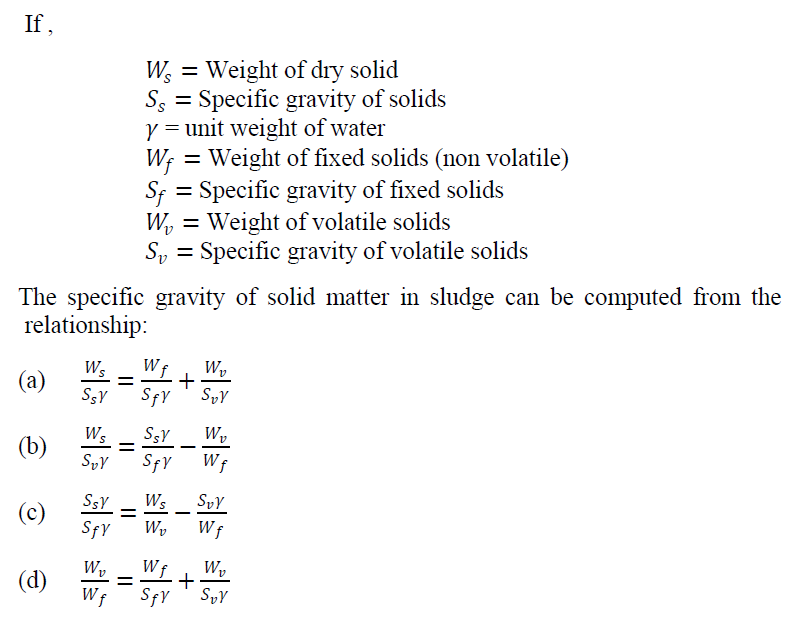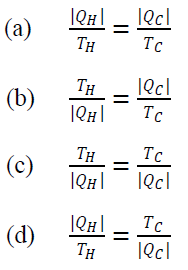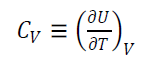### Solved Question Paper for Lecturer in Chemical Engineering in Daman and Diu & Lecturer in Chemical Engineering in DTTE, GNCTD, conducted by UPSC

21. In which one of the following approaches a cubic equation of state is used for both vapour and liquid phases?

(a) Gamma-phi
(b) Phi-phi
(c) Alpha-phi
(d) Beta-phi

22.23. With standard notations Carnot engine can be written as:24. When air is behaving as an ideal gas with heat capacities ratio of 1.4, the molar heat capacity at constant pressure will be:
(a) 29.1 J/mol-K
(b) 28.3 J/mol-K
(c) 27.5 J/mol-K
(d) 26.7 J/mol-K

25. The first law of thermodynamics is also known as:
(a) Constant energy principle
(b) Conservation of energy principle
(c) Conservation of entropy process
(d) Constant entropy process

26. Consider the following constant-volume heat capacity of a substance:What does 𝑈 represent?
(a) Molar External Energy
(b) Specific Internal Energy
(c) Specific External Energy
(d) Potential Energy

27. ‘The entire power plant, consisting of a compression device, a combustion chamber, and a nozzle’, is a:
(a) Carnot engine
(b) Diesel engine
(c) Petrol engine
(d) Jet engine

28. To maintain the temperature of a solution at 261 K, 1000 kJ of heat per minute is continuously removed from it. The surrounding temperature is 288 K. The least amount of power required will be nearly:
(a) 6.9 hp
(b) 4.7 hp
(c) 3.5 hp
(d) 2.3 hp

29. Which one of following theorems holds true for the statement given below?
‘For any closed system formed initially from given masses of prescribed chemical species, the equilibrium state is completely determined when any two independent variables are fixed’.
(a) Gibbs theorem
(b) Duhem’s theorem
(c) Liouville’s theorem
(d) Guldberg theorem

30. Air at 1 bar and 25°𝐶 enters a compressor at low velocity, discharges at 3 bar, and enters a nozzle in which it expands to a final velocity of 600 𝑚/𝑠 at the initial conditions of pressure and temperature. If the work of compression is 240 𝑘J/kg of air, the amount of heat removed during the compression will be:
(a) −90 kJ/kg
(b) −80 kJ/kg
(c) −70 kJ/kg
(d) −60 kJ/kg

Share:

## Featured Post

### UPSC Civil Service Preliminary Paper-1 Previous Year Solved Question Papers

Civil Service Preliminary Paper-1 Previous Year Solved Questions for the year 2019 Civil Service Preliminary Paper-1 Previous Year Solved Qu...Name

Email *

Message *